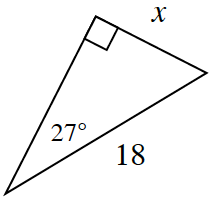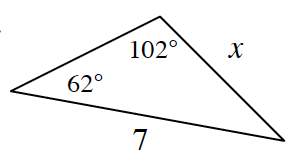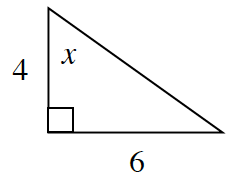### Home > CCG > Chapter 12 > Lesson 12.1.2 > Problem12-25

12-25.

For each diagram below, solve for $x$. Show all work.

1.What trigonometric ratio can you use to find the value of $x$?

$x = 8.17$

1.Use the Law of Sines to find x.

$\frac{ \text{sin}(102^{\circ}) }{7 }= \frac{ \text{sin}(62^{\circ}) }{x }$

$\sin102^\circ(x)=7(\sin62^\circ)$

$x=6.32$

1.What trigonometric ratio can you use to find $x$?